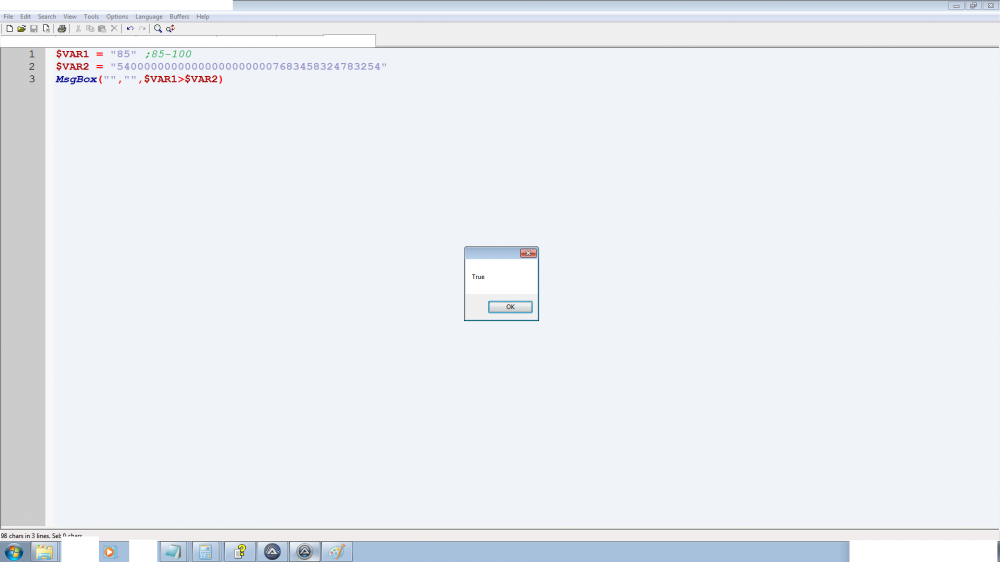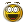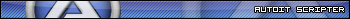# Numeric Strings Comparison

## Recommended Posts

So i am not supposed to check if a numeric string is bigger or smaller than another numeric string? i thought Autoit Converts strings to number and compare them and not compare the Ascii valus or just the first two numbers, i say that because i can do Arithmetic on two numeric strings fine but why cant i compare it as numbers if i can do multiplication and division on it as numbers!

$VAR1 = "85" ;$VAR2 = "6752783465324234658465872365523874652345632456732"
Msgbox("","",$VAR1>$VAR2)Edited by CrypticKiwi
##### Share on other sites

Hello. Strings are compared lexicographically.

Saludos

Edited by DanyfirexDanysys.comAutoIt...

##### Share on other sites

If you don't tell AutoIt how to treat your variable, it decides for you, unfortunately it cannot know for sure.

$VAR1 = "85" ;$VAR2 = "6752783465324234658465872365523874652345632456732"
Msgbox("","",Number($VAR1)>Number($VAR2))

Monkey's are, like, natures humans.

##### Share on other sites

Hello. Strings are compared lexicographically.

Saludos

Thanks Dan, was just trying to understand the logic behind it. i also think that numeric strings comparison should default to comparing them as numbers unless its specified that i want to compare "lexicographically". because i guess that if somebody is trying to compare strings they would want to compare as numbers more than lexicographically in which after checking Wiki for lexicographical Order i still dont understand it.

"For example, the word "Thomas" appears before "Thompson" in dictionaries because the letter 'a' comes before the letter 'p' in the alphabet. The 5th letter is the first that is different in the two words; the first 4 letters are "Thom" in both. Because it is the first difference, the 5th letter is the most significant difference (for an alphabetical ordering)."

i understand this but if i

$Var1 = "Thomas"$Var2 = "Thompson"
Msgbox("","",$Var1>$VAR2) ;is False
Msgbox("","",$Var2>$Var1); is False ;yesterday this Displayed false, today strangely it Displays true!

Maybe its not that simple, anyway thanksEdited by CrypticKiwi
##### Share on other sites

I think it work something like this:

T(84)   T(84)   equal
h(104)  h(104)  equal
o(111)  o(111)  equal
m(109)  m(109)  equal
a(97)   p(112)  Here if you use ">" get false. if you use "<" you get true.
s(115)  s(115)  not compared
(0) o(111)  not compared
(0) n(110)  not compared

SaludosDanysys.comAutoIt...

##### Share on other sites

$VAR2 is way beyond the limits of Int-64. To make a comparison like this you have to roll your own function. The following example only works with integers or longer strings only containing digits. #include <MsgBoxConstants.au3> ; for$MB_OK

Local $VAR1 = "85" Local$VAR2 = "6752783465324234658465872365523874652345632456732"

Msgbox($MB_OK, "", _IsIntGT($VAR1, $VAR2)) Func _IsIntGT($sInt1, $sInt2) ; tests if$sInt1 > $sInt2 If Not (StringIsDigit($sInt1) Or StringIsDigit($sInt2)) Then Return SetError(1) ; integers only$sInt1 = StringRegExpReplace($sInt1, "\A0+", "") ; strip any leading zeros$sInt2 = StringRegExpReplace($sInt2, "\A0+", "") ; ditto Local$iLen1 = StringLen($sInt1),$iLen2 = StringLen($sInt2) ; get the number of digits ; select the appropriate comparison method. Return ($iLen1 = $iLen2) ? StringCompare($sInt1, $sInt2) > 0 :$iLen1 > \$iLen2
EndFunc

##### Share on other sites

Not sure if this is your problem, but please see these entries:

Source From the AutoIT wiki FAQ

## Why are my number sort results wrong?

AutoIt by default uses an alpha sort algorithm. This means that ABC is sorted correctly, but it sorts numbers as 1,10,2,22,3,31 where the user expects 1,2,3,10,22,31. This can be remedied by using a custom sort function. An example of such a custom number sort by Valuater appears below. Search the Forum for Number Sort and Natural Order String Comparison

Skysnake

Why is the snake in the sky?

## Create an account

Register a new account

• ### Recently Browsing   0 members

×

• Wiki

• Back

• #### Beta

• Git
• FAQ
• Our Picks
×
• Create New...